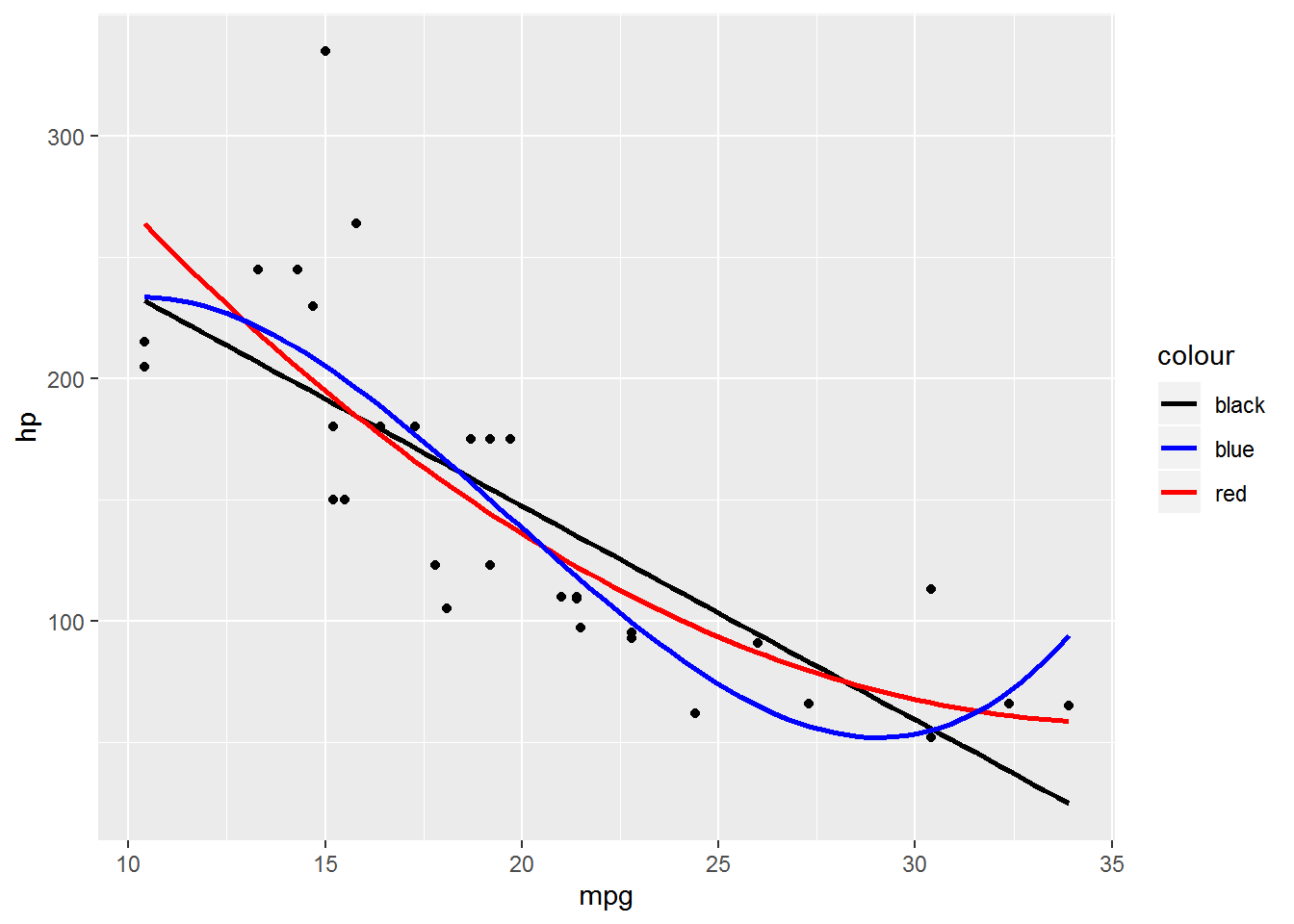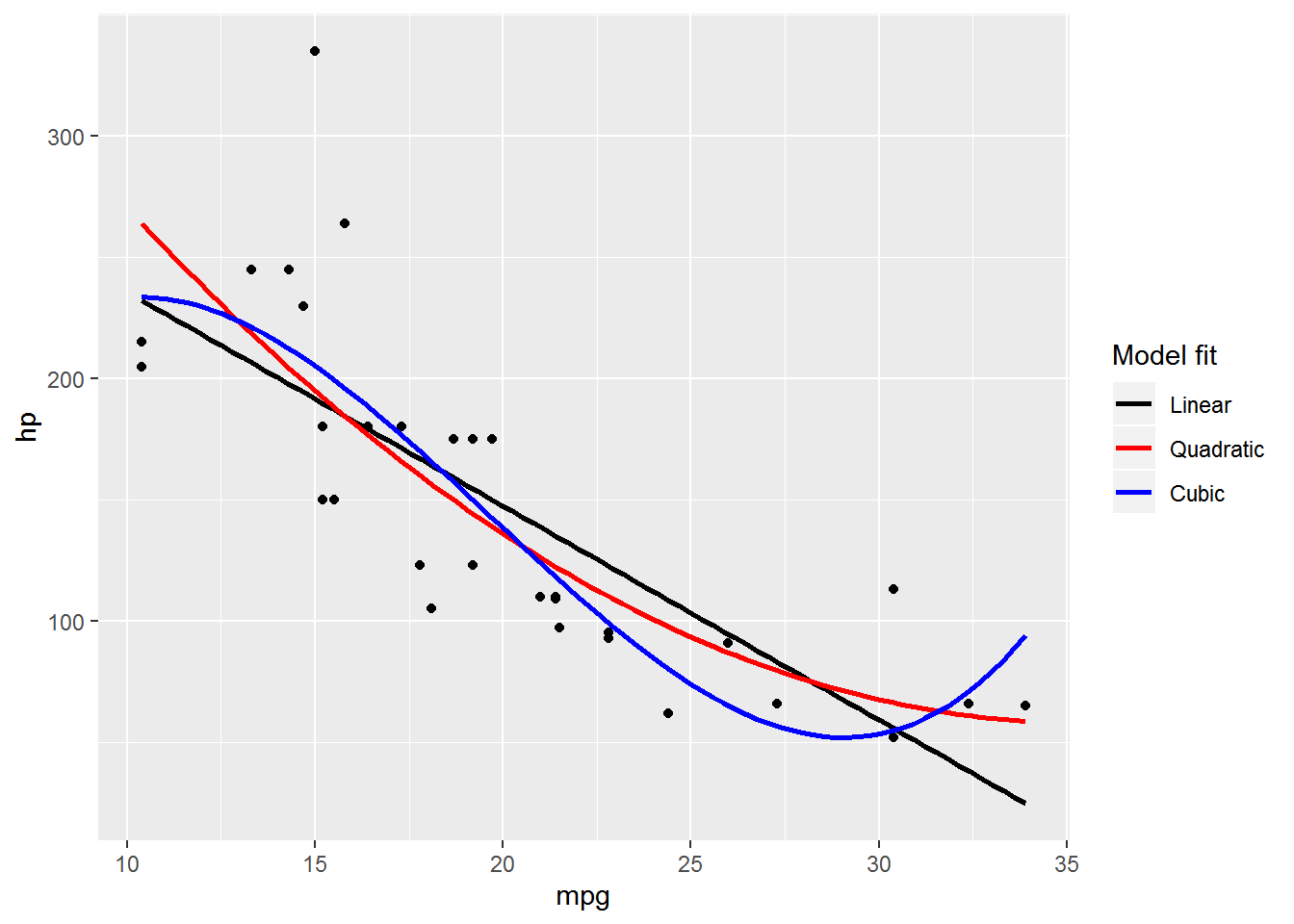Want to share your content on R-bloggers? click here if you have a blog, or here if you don't.

In general, if you want to map an aesthetic to a variable and get a legend in ggplot2 you do it inside `aes()`. If you want to set an aesthetic to a constant value, like making all your points purple, you do it outside `aes()`.

However, there are situations where you might want to set an aesthetic for a layer to a constant but you also want a legend for that aesthetic. One common alternative is to put your dataset into a long format to take advantage of the strengths of ggplot2, but that isn’t a straightforward option for every situation. I’ll show another approach here.

# The setup

A few situations where we might want legends without mapping an aesthetic to a variable are:
1. Adding a statistic like the mean as a line or symbol and wanting a legend to define it
2. Adding separate layers for subsets of data or based on different datasets*
3. Adding lines based on different fitted models

*This second situation is where reformatting your dataset is often most useful

Today I’ll focus on adding lines from different models. I’m going to be using the ubiquitous `mtcars` dataset for the work. 😆

# Making a plot with aesthetics as constants

`library(ggplot2) # v. 3.2.0`

I’m going to make a plot of the relationship between `mpg` and `hp`, adding three fitted lines from three different linear regression models. I will use a linear, a quadratic, and a cubic model. I use `geom_smooth()` to make the fitted regression lines, and so add a separate `geom_smooth()` layer for each model.

I’m going to focus on the `color` aesthetic here, but this is relevant for other aesthetics, as well.

You can see I set a different `color` per fitted line. Since I’m setting these colors as constants this is done outside `aes()`.

```ggplot(mtcars, aes(mpg, hp) ) +
geom_point() +
geom_smooth(method = "lm", se = FALSE, color = "black") +
geom_smooth(method = "lm", formula = y ~ poly(x, 2), se = FALSE, color = "red") +
geom_smooth(method = "lm", formula = y ~ poly(x, 3), se = FALSE, color = "blue")```It would be nice to know which line came from which model, and adding a legend is one way to do that. So how can we do this?

# Why won’t scale_color_manual() make a legend?

I think for many people it feels intuitive to add the appropriate `scale_*()` function to the plotting code in hopes of getting a legend. Along those lines I’ll add `scale_color_manual()` to my plot.

```ggplot(mtcars, aes(mpg, hp) ) +
geom_point() +
geom_smooth(method = "lm", se = FALSE, color = "black") +
geom_smooth(method = "lm", formula = y ~ poly(x, 2), se = FALSE, color = "red") +
geom_smooth(method = "lm", formula = y ~ poly(x, 3), se = FALSE, color = "blue") +
scale_color_manual(values = c("black", "red", "blue") )```But nothing changes. Unfortunately, no matter how hard I throw `scale_color_manual()` at the plot, I won’t get a legend in this way.

Why doesn’t this work?

From the description in the `scale_manual` documentation, the manual scale functions allow you to specify your own set of mappings from levels in the data to aesthetic values. You can change already created mappings but not construct them. In ggplot2, mappings are constructed by `aes()`. Aesthetics therefore must be inside `aes()` to get a legend.

# Adding a legend by moving aesthetics into aes()

I’ll move `color` inside of `aes()` within each `geom_smooth()` layer to construct color mappings. This adds a legend to the plot.

```ggplot(mtcars, aes(mpg, hp) ) +
geom_point() +
geom_smooth(method = "lm", se = FALSE, aes(color = "black") ) +
geom_smooth(method = "lm", formula = y ~ poly(x, 2), se = FALSE, aes(color = "red") ) +
geom_smooth(method = "lm", formula = y ~ poly(x, 3), se = FALSE, aes(color = "blue") )```My plot now has a legend 👍, but the colors have changed 👎. The values are no longer recognized as colors since `aes()` treats these as string constants. To get the desired colors we’ll need to turn to one of the `scale_color_*()` functions.

# Using scale_color_identity() to recognize color strings

One way to force `ggplot` to recognize the color names when they are inside `aes()` is to use `scale_color_identity()`. To get a legend with an identity scale you must use `guide = "legend"`. (The default is `guide = "none"` for identity scales.)

```ggplot(mtcars, aes(mpg, hp) ) +
geom_point() +
geom_smooth(method = "lm", se = FALSE, aes(color = "black") ) +
geom_smooth(method = "lm", formula = y ~ poly(x, 2), se = FALSE, aes(color = "red") ) +
geom_smooth(method = "lm", formula = y ~ poly(x, 3), se = FALSE, aes(color = "blue") ) +
scale_color_identity(guide = "legend")```The colors are now correct but the legend still leaves a lot to be desired. The name of the legend isn’t useful, the order is alphabetical instead of by model complexity, and the labels are the color names instead of descriptive names that describe each model.

The legend name can be changed via `name`, the order can be changes via `breaks` and the labels can be changed via `labels` in `scale_color_identity()`. The order of the `labels` must be the same as the order of the `breaks`.

This all means the `scale_color_identity()` code gets quite a bit more complicated. I’ve found this to be pretty standard when mapping aesthetics to constants.

```ggplot(mtcars, aes(mpg, hp) ) +
geom_point() +
geom_smooth(method = "lm", se = FALSE, aes(color = "black") ) +
geom_smooth(method = "lm", formula = y ~ poly(x, 2), se = FALSE, aes(color = "red") ) +
geom_smooth(method = "lm", formula = y ~ poly(x, 3), se = FALSE, aes(color = "blue") ) +
scale_color_identity(name = "Model fit",
breaks = c("black", "red", "blue"),
guide = "legend")```# Descriptive strings and scale_color_manual()

An alternative (but not necessarily simpler 😄) approach is to use informative string names instead of the color names within `aes()`. Then we can use `scale_color_manual()` to get the legend cleaned up.

Here is the plot using descriptive names that describe each model instead of the color names.

```ggplot(mtcars, aes(mpg, hp) ) +
geom_point() +
geom_smooth(method = "lm", se = FALSE, aes(color = "Linear") ) +
geom_smooth(method = "lm", formula = y ~ poly(x, 2), se = FALSE, aes(color = "Quadratic") ) +
geom_smooth(method = "lm", formula = y ~ poly(x, 3), se = FALSE, aes(color = "Cubic") )```This has nicer labels, but the legend has other problems, similar to those above. The legend name isn’t informative, the order is again alphabetical instead of by model complexity, and the colors still need to be changed if we really want black, red, and blue lines. This can all be addressed in `scale_color_manual()`.

For the first two issues I will again use `name` and `breaks` to get things named and in the desired order.

Colors are set via passing a vector of color names to the `values` argument in `scale_color_manual()`. Note the `values` argument is a required aesthetic in `scale_color_manual()`; if you don’t want to change the colors in the plot use `scale_color_discrete()`.

The vector of colors needs to either be in the same order as the `breaks` or given as a named vector. The latter is “safest” since it is invariant to changing the order of the legend, and so I’ll use a named vector in my example code.

```ggplot(mtcars, aes(mpg, hp) ) +
geom_point() +
geom_smooth(method = "lm", se = FALSE, aes(color = "Linear") ) +
geom_smooth(method = "lm", formula = y ~ poly(x, 2), se = FALSE, aes(color = "Quadratic") ) +
geom_smooth(method = "lm", formula = y ~ poly(x, 3), se = FALSE, aes(color = "Cubic") ) +
scale_color_manual(name = "Model fit",
values = c("Cubic" = "blue", "Quadratic" = "red", "Linear" = "black") )```# Other examples on Stack Overflow

You can see what I would consider some of the canonical questions and answers on this topic from Stack Overflow here and here. (I’m sure there are others, but these are two that I’ve been linking to as duplicates recently. 😺)

Here’s the code without all the discussion. Copy and paste the code below or you can download an R script of uncommented code from here.

```library(ggplot2) # v. 3.2.0

ggplot(mtcars, aes(mpg, hp) ) +
geom_point() +
geom_smooth(method = "lm", se = FALSE, color = "black") +
geom_smooth(method = "lm", formula = y ~ poly(x, 2), se = FALSE, color = "red") +
geom_smooth(method = "lm", formula = y ~ poly(x, 3), se = FALSE, color = "blue")

ggplot(mtcars, aes(mpg, hp) ) +
geom_point() +
geom_smooth(method = "lm", se = FALSE, color = "black") +
geom_smooth(method = "lm", formula = y ~ poly(x, 2), se = FALSE, color = "red") +
geom_smooth(method = "lm", formula = y ~ poly(x, 3), se = FALSE, color = "blue") +
scale_color_manual(values = c("black", "red", "blue") )

ggplot(mtcars, aes(mpg, hp) ) +
geom_point() +
geom_smooth(method = "lm", se = FALSE, aes(color = "black") ) +
geom_smooth(method = "lm", formula = y ~ poly(x, 2), se = FALSE, aes(color = "red") ) +
geom_smooth(method = "lm", formula = y ~ poly(x, 3), se = FALSE, aes(color = "blue") )

ggplot(mtcars, aes(mpg, hp) ) +
geom_point() +
geom_smooth(method = "lm", se = FALSE, aes(color = "black") ) +
geom_smooth(method = "lm", formula = y ~ poly(x, 2), se = FALSE, aes(color = "red") ) +
geom_smooth(method = "lm", formula = y ~ poly(x, 3), se = FALSE, aes(color = "blue") ) +
scale_color_identity(guide = "legend")

ggplot(mtcars, aes(mpg, hp) ) +
geom_point() +
geom_smooth(method = "lm", se = FALSE, aes(color = "black") ) +
geom_smooth(method = "lm", formula = y ~ poly(x, 2), se = FALSE, aes(color = "red") ) +
geom_smooth(method = "lm", formula = y ~ poly(x, 3), se = FALSE, aes(color = "blue") ) +
scale_color_identity(name = "Model fit",
breaks = c("black", "red", "blue"),
guide = "legend")

ggplot(mtcars, aes(mpg, hp) ) +
geom_point() +
geom_smooth(method = "lm", se = FALSE, aes(color = "Linear") ) +
geom_smooth(method = "lm", formula = y ~ poly(x, 2), se = FALSE, aes(color = "Quadratic") ) +
geom_smooth(method = "lm", formula = y ~ poly(x, 3), se = FALSE, aes(color = "Cubic") )

ggplot(mtcars, aes(mpg, hp) ) +
geom_point() +
geom_smooth(method = "lm", se = FALSE, aes(color = "Linear") ) +
geom_smooth(method = "lm", formula = y ~ poly(x, 2), se = FALSE, aes(color = "Quadratic") ) +
geom_smooth(method = "lm", formula = y ~ poly(x, 3), se = FALSE, aes(color = "Cubic") ) +
scale_color_manual(name = "Model fit",